Start typing, then use the up and down arrows to select an option from the list.# Dipole Moment Example 2

Jules Bruno
285views
1
2
for those listed below, which has the most polar bond. Remember, the most polar bond would be attached to the greatest difference and electro negativity. Looking at the electoral negativity is chart. We'd see that sulfur is 2.5. Selenium is 2.4. We'd see here to five. Hydrogen would be to one chlorine. Here would be three point. Oh, and here we have flooring at four point out sulfur again is to five and four inches. 40 This would be 2.5, and this would be 3.5. Remember, difference in electro negativity is the larger electro negativity value minus a smaller one. Here. If we did that before minus 2.5, which would give us 1.5. We see that option D gives us the biggest difference in electro negativity, which would translate into the most polar bond present. So just remember, most polar bond means biggest difference in electro negativity is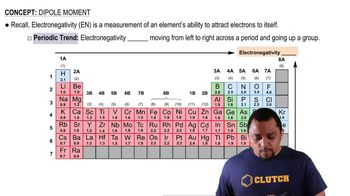01:33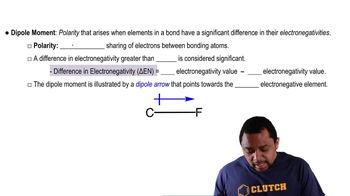01:25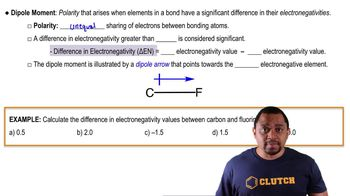02:14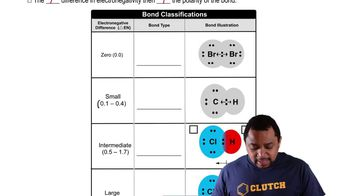03:18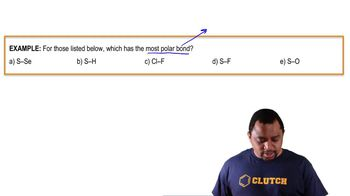01:07